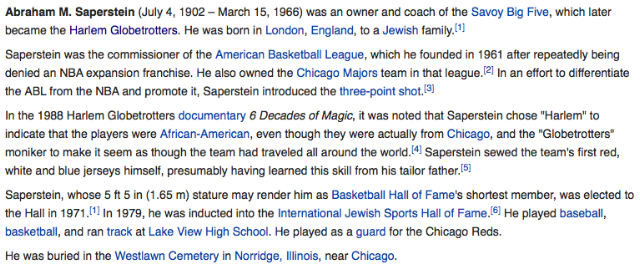## Tuesday, December 29, 2015

### 44 47 54 59 63 74 89 98 113 | The Death of Meadowlark Lemon & Marques Haynes of the Harlem GlobetrottersWilmington, NC = 5+9+3+4+9+5+7+2+6+5+5+3 = 63 (Meadowlark Lemon = 63)Scottsdale, AZ = 37/55; Wilmington = 55
Lemon = 12+5+13+15+14 = 59 (Negro, Slave, Blues) (Globetrotters)

Lemon = 3+5+4+6+5 = 23
Meadowlark Lemon = 63 (Wore #36)

Notice that Lemon was born in North Carolina, what I refer to as 'basketball weirdo land'.  He was born on April 25, 1932, the 116th day of the year, and a date with a life lesson number of '44', sometimes referred to as the 'kill number'.

4/25/1932 = 4+25+19+32 = 80 (Eighty = 5+9+7+8+20+25 = 74)
4/25/1932 = 4+25+(1+9+3+2) = 44
4/25/1932 = 4+2+5+1+9+3+2 = 26 (God = 26) (Globetrotters established in 1926)
4/25/32 = 4+25+32 = 61 (Meadow = 61) (God = 61, Jewish Gematria)

12/27/2015 = 12+27+20+15 = 74
12/27/2015 = 12+27+(2+0+1+5) = 47
12/27/2015 = 1+2+2+7+2+0+1+5 = 20
12/27/15 = 12+27+15 = 54 (He was 22-years old) (Basketball = 22)

Notice the date he died on had numerology of '54', matching the year he joined The Harlem Globetrotters, 1954.  Also, he died 61-years later, in 2015, corresponding with his birth numerology.

The = 20+8+5 = 33
Harlem = 8+1+18+12+5+13 = 57
Globetrotters = 7+12+15+2+5+20+18+15+20+20+5+18+19 = 176
The Harlem Globetrotters = 266
Harlem Globetrotters = 233

The = 2+8+5 = 15
Harlem = 8+1+9+3+5+4 = 30
Globetrotters = 7+3+6+2+5+2+9+6+2+2+5+9+1 = 59/68 (Lemon = 59) (Negro = 59)
The Harlem Globetrotters = 104/113
Harlem Globetrotters = 89/98 (Sweet Georgia Brown = 89/98)

Speak of 89, the Globetrotters are 89-years old this year, 2015, being established in 1926.

https://en.wikipedia.org/wiki/Harlem_Globetrotters

Do you think it is any coincidence the name 'Globetrotters' has gematria synchronicity with 'negro'?  I for one, certainly don't.  Further, do you think it is any coincidence that the name Lemon has matching gematria as well, considering the fact that Lemon was the leader of the Globetrotters?

Notice how they mention Meadowlark Lemon was 6'3", matching the gematria of '63' for his name and place of birth, Wilmington, NC.

Notice that he played basketball with the Globetrotters for 22-years, as the ringmaster.

Master = 4+1+1+2+5+9 = 22/31
Clown = 3+3+6+5+5 = 22 (Clown Prince)
The powers that be love their multiples of 11 (31 is the 11th Prime Number)

The Globetrotters theme song, 'Sweet Georgia Brown', has familiar Gematria, matching 'Harlem Globetrotters'.

Sweet = 1+5+5+5+2 = 18/27
Georgia = 7+5+6+9+7+9+1 = 44
Brown = 2+9+6+5+5 = 27
Sweet Georgia Brown = 89/98 (Harlem Globetrotters = 89/98)

Notice how the article tries to make the distinction that the Globetrotters played fixed games, as if that isn't what is done in the NBA...

The date the Globetrotters record win streak came to an end has familiar numerology.

1/5/1971 = 1+5+19+71 = 96 (Freemason = 96)
1/5/1971 = 1+5+1+9+7+1 = 24
1/5/71 = 1+5+71 = 77

Notice the mention of the death of Saperstein and the team changing hands.  The name of the Jew controlling the team has gematria matching up with Meadowlark Lemon.

Saperstein = 1+1+7+5+9+1+2+5+9+5 = 45/54/63 (Meadowlark Lemon = 63, began career in '54)
Saperstein died at age 63...Notice that Lemon's handler was born on 7/4 and died on the 74th day of the yearJewish = 74; Masonic = 74
Also notice the mention of the death of his teammate, Marques Haynes, this year in May, at age 89, when 'Harlem Globetrotters' has Gematria of 89, as well as 'Sweet Georgia Brown'.

http://www.nytimes.com/2015/05/23/sports/marques-haynes-harlem-globetrotters-star-dribbler-dies-at-89.html

Marques = 13+1+18+17+21+5+19 = 94
Haynes = 8+1+25+14+5+19 = 72
Marques Haynes = 166

Marques = 4+1+9+8+3+5+1 = 31/40
Haynes = 8+1+7+5+5+1 = 27/36
Marques Haynes= 58/76

3/10/1926 = 3+10+19+26 = 58
3/10/1926 = 3+10+(1+9+2+6) = 31
3/10/1926 = 3+1+0+1+9+2+6 = 22
3/10/26 = 3+10+26 = 39

5/22/2015 = 5+22+20+15 = 62
5/22/2015 = 5+22+(2+0+1+5) = 35
5/22/2015 = 5+2+2+2+0+1+5 = 17
5/22/15 = 5+22+15 = 42

As it happens, May 22 is the 142nd day of the year, and there is very much a relationship between 42 and 142, especially for black americans, which Marques Haynes was.

Forty-Two = 6+15+18+20+25+20+23+15 = 142

It is also the day that leaves 223 days remaining in the year, a very Masonic number.  Looking at the facts, I don't think it is any coincidence he died at age 89...  Also, let us not forget that Meadowlark Lemon died on the 33rd Parallel, in Scottsdale, AZ, when '33' is the number of obsession and sacrifice for the Masons.

Sweet = 1+5+5+5+2 = 18/27
Georgia = 7+5+6+9+7+9+1 = 44
Brown = 2+9+6+5+5 = 27
Sweet Georgia Brown = 89/98 (Harlem Globetrotters = 89/98)

Harlem = 8+1+9+3+5+4 = 30
Globetrotters = 7+3+6+2+5+2+9+6+2+2+5+9+1 = 59/68 (Negro = 59)
Harlem Globetrotters = 89/98